Poor control loop stability results in degraded power supply rejection ratio, transient response, output noise, and, for mixed signal circuits, degraded jitter. Datasheet application examples and reference designs do not often include stability information, and measurements often conclude the stability is poor.

One method of designing stable control loops is to create an accurate broadband simulation model and to use pole zero compensation to optimize phase margin, gain margin and stability margin.

This is a time-consuming process and much of the data needed to create an accurate model is unpublished. Another issue is that many circuits are completely integrated without access to the control loops for measurement. Information on stability performance in data sheets and reference design literature is often limited or non-existent.

This article explains a shortcut to stabilizing a control loop in under 5 minutes without requiring any knowledge of the part’s internal circuitry.

### Stability Assessment

There are well-established methods of assessing stability of black box systems. One method is impedance-based stability, which is popular for assessing the stability of switching converters combined with input filters.1

Another method of assessing stability is the non-invasive stability margin, which is an impedance-based mathematical conversion algorithm that I developed and is available from Picotest for many modern vector network analyzers (VNA).

Opamp manufacturers generally provide an isolation resistance vs. load capacitance curve. This is an indirect way of assuring stability based on their knowledge of the opamp output impedance.2

All these assessments work on the same principle and compute stability from the interaction of impedances between two elements. Once we define stability, we can then use the principles of the above referenced assessment methods, in reverse, to design a stable solution. RF engineers use this method for designing oscillators by defining stability as zero and solving for the negative resistance required to exactly counteract the real resistance of a crystal. Basing our solution on these well-proven and well-published assessment methods puts us on solid ground.

### Equivalent Circuit

Voltage output devices, including opamps, voltage references, and both switching and linear regulators, appear inductive when looking at their outputs. The equivalent circuit is a voltage source in series with a resistor and inductor. The destabilizing load appears as a capacitor with equivalent series resistance (ESR). Current regulators also appear as a L-C resonance circuit. In this case, the current regulator is capacitive. The current regulator’s destabilizing load appears as an inductor with a series resistance. An equivalent circuit supporting both current regulators and voltage regulators is shown in Figure 1.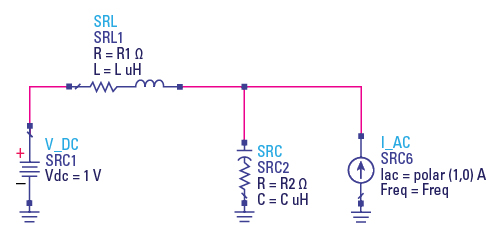Figure 1. Equivalent circuit showing the inductance and capacitance relating to the voltage regulator inductance, current regulator capacitance, and the reactive loading.

In this simulation, L is set to 1 uH and C to 1 uF. This choice normalizes the characteristic impedance of the resonance at 1Ω.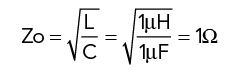The circuit in Figure 1 is simulated while sweeping the resistor R1 and then separately sweeping the resistor R2. The results, shown in Figure 2, show a minima when R1 or R2 are equal to 1.22. And since this is normalized, R1 or R2 would be set to 1.21*Zo.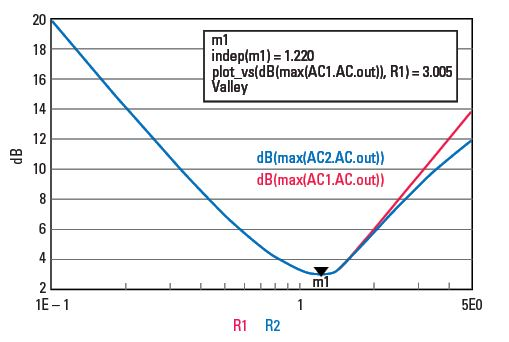Figure 2. The minimum impedance occurs when the total series resistance is 1.22* the characteristic impedance.

Two other cases worth noting are shown in Figure 3. One case where each resistor is set to half of 1.22 or 0.61Ω each, resulting in the red trace (1.1Ω pk). A second case, where each resistor is set to match the 1Ω characteristic impedance, results in flat impedance (1Ω pk). This is the basic principal of connecting 50Ω RF sources to 50Ω RF loads using 50Ω characteristic impedance cable.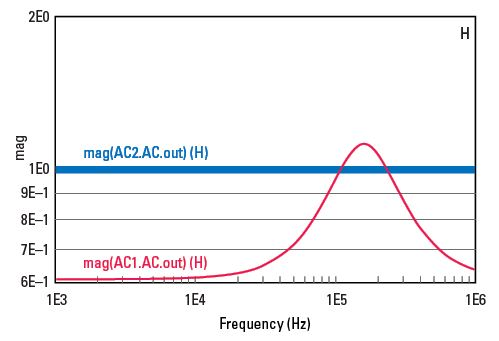Figure 3. There is a certain dependency on where the resistor is located. Note there is a special case where the series resistor and the ESR both match the characteristic impedance (sqrt(L/C)) resulting in flat impedance. With the resistors equally distributed between ESR and series resistance, the peak impedance is 10 percent above the characteristic impedance.

We can apply this knowledge to stabilize any control loop in just a few minutes. We only need to determine the source (opamp, reference, voltage regulator) output inductance value. The fastest way to determine this is by measuring the impedance, either with or without the destabilizing load. You might have several options depending on the VNA you use for the measurement.

An example measurement, performed on a DC-DC converter, is shown in Figure 4. The OMICRON Lab Bode 100 can display the inductance directly; it can be estimated from the impedance 3 dB point, shown in cursor 1, or from the 157 kHz resonant frequency with the 15 uF installed capacitor.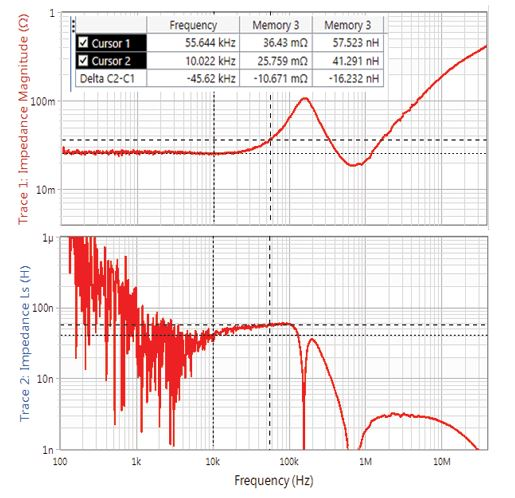Figure 4. The inductance of the voltage regulator can be obtained in a multitude of ways, depending on the VNA used. The Bode 100 used for this example can display it directly, which is preferred. It can also be approximated by the resonant frequency with a capacitor, or from the impedance 3 dB point as noted with the cursors.

Once the inductance is determined, set the total resistance to 1.4* the characteristic impedance. This value varies from 1 to 1.4 depending on the precise circuit but using 1.4 will always be a good choice.

The total resistance can be solved as: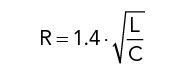In the case of voltage regulators and voltage references, the capacitance and the ESR can both be selected, so there are many choices. Larger capacitors will result in lower maximum impedance. The voltage regulator output impedance from the circuit in Figure 4 is 57 nH and 26 mΩ. The capacitor is 15 uF with an ESR of 10 mΩ. This can be determined from the datasheet, assuming it specifies ESR, but it is more accurately seen from the impedance minima at 700 kHz, in Figure 3, in the impedance measurement.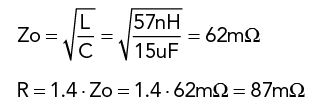The series resistance is 26 mΩ, which can be subtracted from the total resistance required: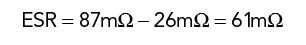The equivalent circuit from Figure 4 is simulated using the 10 mΩ measured capacitor ESR with the calculated 61 mΩ ESR. The results, shown in Figure 5, confirm that setting the ESR to 61 mΩ reduced the maximum at resonance to less than 10 percent above the calculated 62 mΩ characteristic impedance.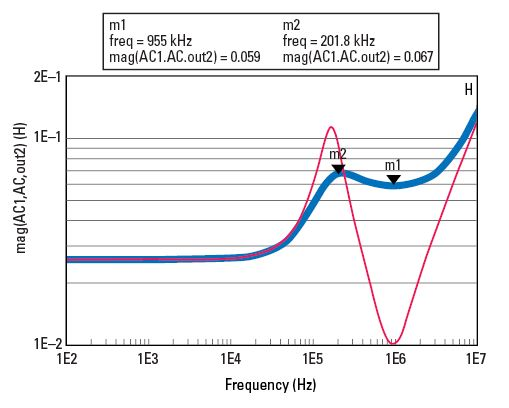Figure 5. A simulation using the inductance and series resistance from the measurement of Figure 4, along with the load capacitor, is simulated here in the RED trace. Setting the capacitor ESR as directed here results in 10 percent peaking above the characteristic impedance, which is a good balance between stability and performance.

### Summary

This method of stabilizing the control loop requires only one quick measurement and is simple to apply to all types of circuits.

1. Determine the inductance of the voltage regulator circuit or capacitance of the current regulator by measuring it directly

2. If the capacitor is defined, solve for the resistor

3. If the capacitor is not defined, select it based on the desired characteristic impedance

4. Set the capacitor ESR to 1.4*Zo—series resistance

The stability can then be confirmed using a traditional Bode plot, NISM, or step load response.

The example I used here is available for free from the Keysight “How To” video channel.4 This full simulation model (see Figure 6) allows direct simulation of the Bode plot for the two cases shown in Figure 5 (see Figures 7 and 8).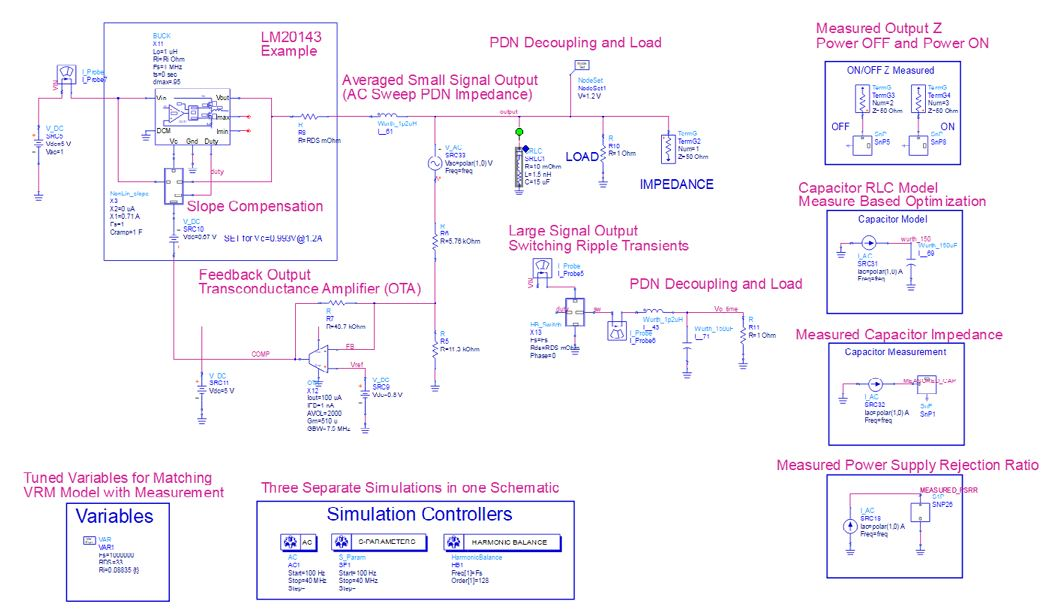Figure 6. Schematic of the LM20143 example DC-DC converter from the Keysight EEs of Power Integrity video channel.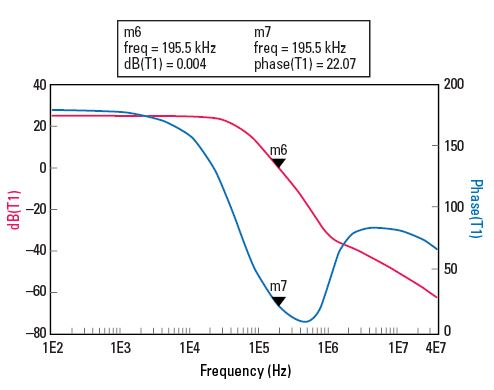Figure 7. The simulated Bode plot using the 15 uF 10 mΩ capacitor shows a phase margin of 22 degrees, which would be considered very poor. This is the cause of the impedance peak seen in Figure 4.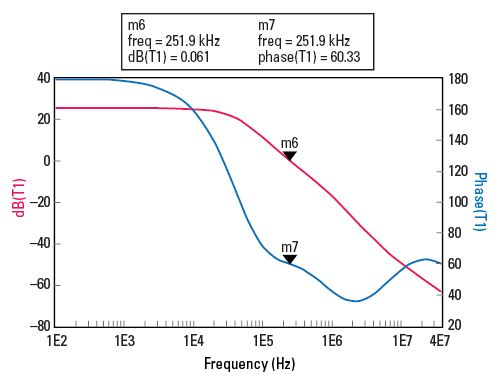Figure 8. The simulated Bode plot using the 15 uF capacitor, but with the 61 mΩ ESR, determined from the proposed method, improved the phase margin from 20 to 60 degrees. 60 degrees is generally seen as the optimum balance between stability and performance.

### Tips

Be sure to measure the active circuit inductance or capacitance at several operating conditions. Many circuits will vary with operating voltage or operating current.

It is a good idea to measure many devices before selecting one for your circuit. The active circuit inductance or capacitance can vary drastically by manufacturer and/or by part. The lower the capacitance of the current regulator and the lower the inductance of the voltage regulator, the better. These reduce the characteristic impedance, requiring less series resistance for stability.

There are two relationships you might find helpful:

1. The output inductance of a switching regulator is not the same as the output filter inductance, but it is proportional to the filter inductance. Reducing the filter inductance value will result in a proportional reduction in the output impedance used for stability assessment.

2. The inductance of a linear regulator, voltage reference, or opamp is inversely proportional to the operating current. Adding a load resistor can greatly reduce the inductance presented, resulting in smaller capacitors. This is particularly true for circuits that can operate at very low output current.

### References

1. C. M. Wildrick, F. C. Lee, B. H. Cho, and B. Choi, “A Method of Defining the Load Impedance Specification for a Stable Distributed Power System,” IEEE Transactions on Power Electronics, Vol. 10, No. 3 , May 1995, DOI: 10.1109/63.387992.

2. P. Semig and T. Claycomb, “Capacitive Load Drive Solution Using an Isolation Resistor,” Texas Instruments, December 2014, www.ti.com/lit/ug/tidu032c/tidu032c.pdf.

3. S. Sandler, “The Inductive Nature of Voltage-Control Loops,” EDN Network, Feburary 5, 2015, www.edn.com/electronics-blogs/impedance-measurement-rescues/4438578/The-inductive-nature-of-voltage-control-loops.

4. “How To Design for Power Integrity: DC-DC Converter Simulation and Modeling,” Keysight EEsof EDA, April 7, 2017, www.youtube.com/watch?v=CNyi4XU9xpY&list=PLtq84kH8xZ9FNXAsf-odoGNe6h5A6D3in&index=6&t=34s.